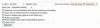By
Nov 04, 2007 (Edited Mar 10, 2014)

Since fields are template variables, one way to add a template variable is simply to add a field. Sometimes you may want to do something more complex, though. All methods of each class are available as template variables, but adding a method to a class isn't upgradable, you'd be stuck with lots of work on every upgrade. As a solution, here's an upgradable way to accomplish the same thing.

The quickest way to describe is to illustrate. Save this as a modifications directory file (say, modifications/example.php):
<?php
\$pseudomethods[] = 'ismine';
\$pseudomethods[] = 'specialformula';

function ismine(\$object)
{ // determine if the object is owned by the viewing member
global \$thismember;
\$r = 0;
if (\$object->ownerid == \$thismember->id && \$thismember->isregistered()) \$r = 1;
return \$r;
}

function specialformula(\$object)
{
{
if (\$object->recipurl && \$object->recipurl != 'http://') \$recipformula = 0.5;
else \$recipformula = -0.5;
\$formula = (((((\$object->numcomments + 2)/(\$object->numcomments + 1)) + ((\$object->hitsin + 10)/(\$object->hitsin + 1)) + (\$object->rating / 2) + \$recipformula) + \$object->pagerank) / 2);
\$r = \$formula;
}
else if (\$object->objecttype == 'member')
{
\$formula = (\$this->submissions() * 2) + \$this->postcount();
if (\$this->time < time() - (86400*100)) \$formula = \$formula * 1.75; // extra credit for sticking around more than 100 days
\$r = \$formula;
}
return \$r;
}
?>

This has added several template variables: {LINKISMINE} (plus {CAT/{COMMENT/{MEMBER versions) and {LINKSPECIALFORMULA} / {MEMBERSPECIALFORMULA}. It's important to note that these functions can apply to all types of objects, so you could actually use {EVENTFUNDSDAYS} as well, though it doesn't make any logical sense so you wouldn't.

Basically all there is to do is write the function, and then add the function name to the \$pseudomethods array as shown at the top.

\$object will be whatever sort of object it's a template variable for, so to work with multiple types of objects you test \$object->objecttype as shown in the example.

The specialformula function is an example for when you have formulas in mind too complicated to do in the listing importance or member rating formula boxes. It provides an illustration of how you test for what type of object you're working with.

Please be careful to ensure that your function name doesn't conflict with any existing object methods, fields or functions. Make it unique. One way to ensure that in a future-proof manner is to use your own prefix, like yourname_functionname.

 Description Like adding methods to the classes, but upgradeable. Rating 0/512345 Views 954 views. Averaging 0 views per day. Similar ListingsAdding a New Field